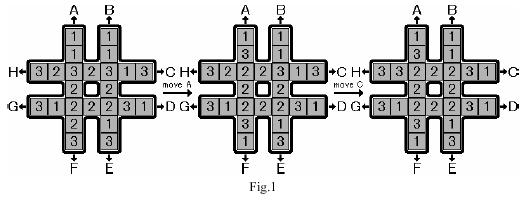# IDDFS（迭代加深搜索）基础题两则

### POJ 3134 - Power Calculus

 1 #include <iostream>
2 #include <cstdlib>
3 #include <cstdio>
4 #include <cstring>
5 #include <string>
6 #include <sstream>
7 #include <algorithm>
8 #include <cmath>
9 #include <vector>
10 #include <stack>
11 #include <queue>
12 #include <list>
13 #define the_best_pony "Rainbow Dash"
14
15 using namespace std;
16
17 int n,maxh;
18 int a;
19
20 bool dfs(int x,int cur){
21     if(x<<(maxh-cur)<n) return false; //乐观估计剪枝，当前深度到限制深度指数最多增长2^(maxh-cur)倍
22     if(cur>maxh) return false; //达到限制深度
23     a[cur]=x;
24     if(x==n) return true;
25     for(int i=0;i<=cur;i++){
26         if(dfs(x+a[i],cur+1)) return true;
27         if(dfs(x>a[i]?x-a[i]:a[i]-x,cur+1)) return true;
28     }
29     return false;
30 }
31
32 int main(){
33     while(scanf("%d",&n)&&n){
34         maxh=0;
35         memset(a,0,sizeof(a));
36         while(!dfs(1,0)){ //只要没找到答案就一直继续
37             memset(a,0,sizeof(a));
38             maxh++; //增大深度限制
39         }
40         printf("%d\n",maxh); //最大深度就是答案
41     }
42     return 0;
43 }

### POJ 2286 - The Rotation Game1 #include <iostream>
2 #include <cstdlib>
3 #include <cstdio>
4 #include <cstring>
5 #include <string>
6 #include <sstream>
7 #include <algorithm>
8 #include <cmath>
9 #include <vector>
10 #include <stack>
11 #include <queue>
12 #include <list>
13 #define the_best_pony "Rainbow Dash"
14
15 using namespace std;
16
17 int rot={                //操作打表
18     {0,2,6,11,15,20,22},         //A
19     {1,3,8,12,17,21,23},         //B
20     {10,9,8,7,6,5,4},            //C
21     {19,18,17,16,15,14,13},     //D
22     {23,21,17,12,8,3,1},        //E
23     {22,20,15,11,6,2,0},         //F
24     {13,14,15,16,17,18,19},     //G
25     {4,5,6,7,8,9,10}             //H
26 };
27 int cent={6,7,8,11,12,15,16,17}; //中间8个位置
28 int a,maxh=1;
29 char put;
30
31 bool check(){ //判断中间是否一样
32     for(int i=0;i<8;i++)
33         if(a[cent]!=a[cent[i]]) return false;
34     return true;
35 }
36
37 int cnt(){
38     int num=~0u>>1;
39     for(int i=1;i<=3;i++){
40         int tmp=0;
41         for(int j=0;j<8;j++)
42             if(a[cent[j]]!=i) tmp++;
43         num=min(num,tmp);
44     }
45     return num;
46 }
47
48 void move(int x){ //操作
49     int tmp=a[rot[x]];
50     for(int i=0;i<6;i++) a[rot[x][i]]=a[rot[x][i+1]];
51     a[rot[x]]=tmp;
52     return;
53 }
54
55 bool dfs(int dep){
56     if(dep>maxh) return false;
57     if(check()){
58         put[dep]='\0';
59         return true;
60     }
61     if(dep+cnt()>maxh) return false; //乐观估计剪枝
62     for(int i=0;i<8;i++){
63         put[dep]='A'+i;
64         move(i);
65         if(dfs(dep+1)) return true;
66         if(i%2==0) move((i+5)%8);     //反向操作
67         else move((i+3)%8);        //反向操作
68     }
69     return false;
70 }
71
72 int main(){
73     while(scanf("%d",&a)&&a){
74         maxh=1;
75         for(int i=1;i<24;i++) scanf("%d",&a[i]);
76         if(check()) printf("No moves needed\n%d\n",a[cent]);
77         else{
78             while(!dfs(0)) maxh++;
79             printf("%s\n%d\n",put,a[cent]);
80         }
81     }
82     return 0;
83 }

posted @ 2017-08-30 12:20  VKorpela  阅读(3377)  评论(3编辑  收藏